# Texas Go Math Grade 5 Lesson 9.2 Answer Key Formulas for Volume

Refer to our Texas Go Math Grade 5 Answer Key Pdf to score good marks in the exams. Test yourself by practicing the problems from Texas Go Math Grade 5 Lesson 9.2 Answer Key Formulas for Volume.

## Texas Go Math Grade 5 Lesson 9.2 Answer Key Formulas for Volume

Unlock the Problem

Margo is modeling a building using 1-centimeter cubes.
The model has a rectangular base and a height of the 5 cubes.
What is the volume of the rectangular prism that Margo built?Use centimeter cubes to show Margo’s model.
Count the total number of cubes after each layer.
Use the information to complete the table.• What multiplication pattern do the numbers in the table show?
________v= 8 X h_____________
• Why do we multiply by 8?
_______because the cubic has 8 edges,______________Multiplication pattern does the numbers in the table show are v = 8 X h,
We multiply by 8 because cubic has 8 edges,

Explanation:
Counting the total number of cubes after each layer.
Using the information and completed the table as shown above,
Multiplication pattern does the numbers in the table shown are v = 8 X h
where h is height in layers and v is volume in cubic centimeters,
if h =1 then v = 8 X 1 = 8,
if h = 2 then v = 8 X 2 = 16,
if h = 3 then v = 8 X 3 = 24,
if h = 4 then v = 8 X 4 =32 and
if h = 5 then v = 8  X 5 = 40,

You can find the volume of a prism in cubic units by multiplying the
number of square units in the base shape by the number of layers, or its height.
• Write a formula for finding the volume of a rectangular prism.
Use B for the area of the base and h for the height.
________v =B X h_____________
So, the volume of Margo’s rectangular prism is ____Bh_______ cubic centimeters.
v = Bh,

Explanation:
For finding the volume of a rectangular prism we use
B for the area of the base and h for the height
So, the volume of Margo’s rectangular prism is B X h cubic centimeters.

Relate Height to Volume

You can use the formula for the area of a rectangle to rewrite the
formula for the volume of a rectangular prism.
• What are the dimensions of the base of the box?
_____________________
• What operation can you use to find the area of the base shape?
_____________________
The dimensions of the base of the box is b,
We use multiplication to find the area of the base shape as
a or v= Bh,

Explanation:
The formula for the area of a rectangle if  B is breadth and length is h
which is similar to volume of a rectangular prism with Base B and height h
is a or v = Bh, where the dimensions of the base of the box is b,
used multiplication to find the area of the base shape as a or v= Bh.

Toni stacks cube-shaped beads that measure 1 centimeter on each edge in a storage box.
The box can hold 6 layers of 24 cubes with no gaps or overlaps.
What is the volume of Toni’s storage box?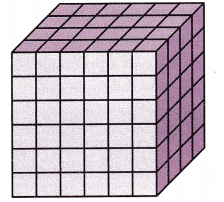One Way
Use V = Bh.
The volume of each bead is ___1______ cubic cm.
The storage box has a base with an area of ____6_____ square cm.
The height of the storage box is _____24____ centimeters.
The volume of the storage box is
Base area (____6_____ × ___24______), or __144__ cubic cm.

Another Way
Use length, width, and height.
The base is a rectangle. Replace B with an expression for the area of the base shape.
V = B × h, B = base area; l = length; W = width
V = Base area (_____6____ × ___24______) × h
The base has a length of ______6______ centimeters and a width of _____24____ centimeters.
The height is ____1_______ centimeters.
The volume of the storage box is ( _____6___ × ____24____) × ____1____, or __6______ × ____24____, or ___144_____ cubic cm.
So, the volume of Toni’s storage box is _____144____ cubic cm.
The volume of storage box is 144 cubic cm,

Explanation:
One Way is using V = Bh.
Given the volume of each bead is 1 cubic cm.
The storage box has a base with an area of 6 square cm.
The height of the storage box is 24 centimeters.
The volume of the storage box is Base area 6 X 24, or 144 cubic cm,
Another Way is Using length, width, and height
The base is a rectangle. Replace B with an expression for the area of the base shape.
V = B × h, B = base area; l = length; W = width
V = 6 X 24 × h
The base has a length of 6 centimeters and a width of 24 centimeters,
The height is 1 centimeters.
The volume of the storage box is 6 X 24 X 1 or 6 X 24 or 144 cubic cm.
So, the volume of Toni’s storage box is 144 cubic cm.

Share and Show

Find the volume.

Question 1.
The length of the rectangular prism is ____5 in________.
The width is ___4 in_________ . So, the area of the base is ___20 square inches_________.
The height is _____6 in_______. So, the volume of the prism is ___120 cubic inches_________.The area of the base is 20 square inches,
The volume of the prism is 120 cubic inches,

Explanation:
Given the length of the rectangular prism is 5 in,
the width is 4 in. So, the area of the base is 5 X 4 =20 square inches.
and the height is 6 in. So, the volume of the prism is l  X w X h =
5 in X 4 in  X 6 in = 120 cubic inches.

Question 2.Volume: _____18 cubic cm________
Volume is 18 cubic cm,

Explanation:
Given the length of the rectangular prism is 2 cm,
the width is 3 cm and the height is 3 cm.
So, the volume of the prism is l  X w X h =
2 cm X 3 cm X 3 cm = 18 cubic cm.

Question 3.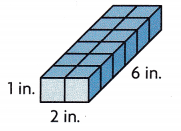Volume: _____12 cubic inches_________
Volume is 12 cubic inches,

Explanation:
Given the length of the rectangular prism is 2 in,
the width is 6 in and the height is 1 in.
So, the volume of the prism is l  X w X h =
2 in X 6 in X 1 in = 12 cubic inches.

Problem Solving

Question 4.
H.O.T. Connect What happens to the volume of a rectangular prism
if you double the height? Give an example.
The volume of a rectangular prism increases,

Explanation:
Let us take the length of the rectangular prism is 5 in,
the width is 3 in and the height is 2 in.
So, the volume of the prism is l  X w X h =
5 in X 3 in X 2 in = 30 cubic inches now if we double
the height from 2 in to 4 in the volume of the prism will
become 5 in X 3 in X 4 in = 60 cubic inches,
so the volume of a rectangular prism increased from 30 cubic inches
to 60 cubic inches.

Question 5.
H.O.T. Write Math Explain how the two formulas for the
volume of a rectangular prism V = Bh and V = l × w × h are related.
Use a model with centimeter cubes to justify your reasoning.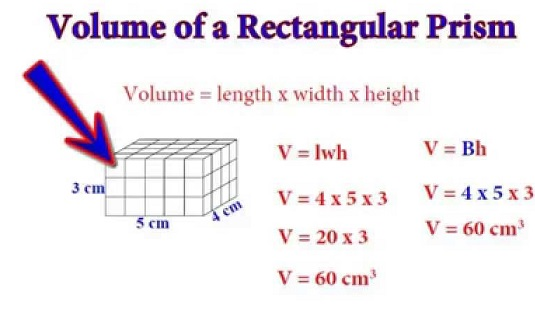Explanation:
The two formulas for the volume of a rectangular prism are
V = Bh and V = l × w × h as l X w = area of base B
so both formulas are related, for example let us take
the length of the rectangular prism is 4 cm,
the width is 5 cm and the height is 3 cm.
So, the volume of the prism is l X w X h = 4 X 5 X 3 = 60 cubic cm as
we know Base area B = l X w so we substitute for
volume of the prism is (l X w ) X h = 20 square cm X 3 cm = 60 cubic cm,
therefore volume of the prism is lwh or Bh as B = lh both are related.

Problem Solving

Question 6.
H.O.T. Multi-Step Rich is building a travel crate for his dog,
Thomas, a beagle-mix who is about 30 inches long, 12 inches wide, and
24 inches tall. For Thomas to travel safely, his crate needs to be a
rectangular prism that is about 12 inches greater than his length and width, and
6 inches greater than his height. What is the volume of the travel crate that Rich should build?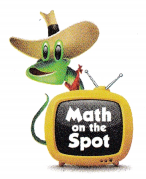The volume of the travel crate that Rich should build is

Explanation:
Given Rich is building a travel crate for his dog,
Thomas, a beagle-mix who is about 30 inches long, 12 inches wide, and
24 inches tall. For Thomas to travel safely, his crate needs to be a
rectangular prism that is about 12 inches greater than his length and width, and
6 inches greater than his height so the crate has length = 30 + 12 = 42 inches,
width = 12 + 12 = 24 inches and height 24 + 6 = 30 inches,
so the volume of the travel crate that Rich should build 42 X 24 X 30 = 30,240 cubic inches.

Question 7.
stacked in layers, with the same number of beads in each layer.
Each layer is 12 beads across and 7 beads wide. There are 4 layers.

Explanation:
Given Sandra orders a box of cube-shaped beads. The beads are neatly
stacked in layers, with the same number of beads in each layer.
Each layer is 12 beads across and 7 beads wide. There are 4 layers.
which matches with (B).

Question 8.
Which equation can you use to find the number of cubes in the rectangular prism?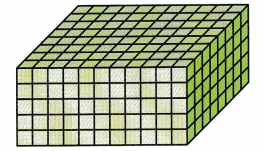(A) V = 11 × 8 × 5
(B) V = 11 + 8 + 5
(C) V = 11 × 8 + 5
(D) V = 2(11 × 8 × 5)
(A) V = 11 × 8 × 5,

Explanation:
To find the number of cubes in the rectangular prism as we
have V = length X width X height the prism has length 11, width 8 and
height 5 so the equation we can use is V = 11 × 8 × 5 matches with (A).

Question 9.
Multi-Step How many cubic units of material were used to make the two rectangular prisms?(A) 144 cubic units
(B) 8,640 cubic units
(C) 60 cubic units
(D) 204 cubic units
(D) 204 cubic units,

Explanation:
Given two rectangular prisms with one prism has length 8, width 6 and
height 3 so 1 prism used 8 X 6 X 3 = 144 cubic units other prism has
length 6 width 5 and  height 2 so 2 prism used 6 X 5 X 2 = 60 cubic units,
So total cubic units of material were used to make the two rectangular prisms are
144 + 60 = 204 cubic units matches with (D).

Texas Test Prep

Question 10.
What is the volume of the rectangular prism at the right?(A) 125 cu in.
(B) 35 cu in.
(C) 155 cu in.
(D) 175 cu in.
(D) 175 cu in,

Explanation:
The given rectangular prism has length 5 in, width 7 in and
height 5 in so the volume of the rectangular prism is 5 in × 7 in × 5 in =
175 cu in matches with (D).

### Texas Go Math Grade 5 Lesson 9.2 Homework and Practice Answer Key

Find the volume.

Question 1.Volume: _______12 cubic cm_______
Volume is 12 cubic cm,

Explanation:
The given rectangular prism has length 3 cm, width 2 cm and
height 2 cm so the volume of the rectangular prism is 3 cm × 2 cm × 2 cm = 12 cubic cm.

Question 2.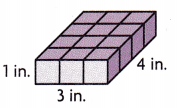Volume: ____12 cubic in__________
Volume is 12 cubic in,

Explanation:
The given rectangular prism has length 3 in, width 4 in and
height 1 in so the volume of the rectangular prism is 3 in × 4 in × 1 in = 12 cubic in.

Question 3.Volume: _____90 cubic cm_________
Volume is 90 cubic cm,

Explanation:
The given rectangular prism has length 6 cm, width 3 cm and
height 5 cm so the volume of the rectangular prism is 6 cm × 3 cm × 5 cm = 90 cubic cm.

Question 4.Volume: ______125 cubic in________
Volume is 125 cubic in,

Explanation:
The given rectangular prism has length 5 in, width 5 in and
height 5 in so the volume of the rectangular prism is 5 in × 5 in × 5 in = 125 cubic in.

Question 5.Volume: _____72 cubic cm_________
Volume is 72 cubic cm,

Explanation:
The given rectangular prism has length 4 cm, width 6 cm and
height 3 cm so the volume of the rectangular prism is 4 cm × 6 cm × 3 cm = 72 cubic cm.

Question 6.Volume: ____36 cubic in__________
Volume is 36 cubic in,

Explanation:
The given rectangular prism has length 3 in, width 3 in and
height 4 in so the volume of the rectangular prism is 3 in × 3 in × 4 in = 36 cubic in.

Problem Solving

Question 7.
A small refrigerator fits into a cabinet that measures 2 feet wide, 2 feet deep, and
4 feet high. What is the volume of the cabinet?
The volume of the cabinet is 16 cubic feet,

Explanation:
Given a small refrigerator fits into a cabinet that measures 2 feet wide, 2 feet deep, and
4 feet high. So the volume of the cabinet is 2 feet X  2 feet X 4 feet = 16 cubic feet.

Question 8.
Mr. Otis built a storage shed. The shed has a length of 5 meters,
a width of 3 meters, and a height of 4 meters. His goal was for the
shed to have a volume greater than 50 cubic meters.
Did Mr. Otis meet his goal? Explain.
Yes, Mr. Otis meet his goal,

Explanation:
Given Mr. Otis built a storage shed. The shed has a length of 5 meters,
a width of 3 meters, and a height of 4 meters the volume of the shed is
5 X 3 X 4 = 60 cubic meters, As Mr. Otis goal was for the shed to have a
volume greater than 50 cubic meters and 60 cubic meters is greater than
50 cubic meters, therefore Mr. Otis will meet his goal.

Lesson Check

Question 9.
What is the volume of the rectangular prism shown below?(A) 48 cu cm
(B) 84 cu cm
(C) 288 cu cm
(D) 384 cu cm
(C) 288 cu cm,

Explanation:
The given rectangular prism has length 6 cm, width 8 cm and
height 6 cm, so the volume of the rectangular prism is
6 cm × 8 cm × 6 cm = 288 cubic cm which matches with (C).

Question 10.
Hannah used centimeter cubes to build the model shown below.Which equation can you use to find the volume of Hannah’s model?
(A) V = 6 + 7 + 3
(B) V = (6 × 7) + 3
(C) V = (6 + 7) × 3
(D) V = 6 × 7 × 3
(D) V = 6 × 7 × 3,

Explanation:
To find the volume of Hannah used centimeter cubes to build the model as we
have V = length X width X height the model has length 6, width 7 and
height 3 so the equation we can use is V = 6 × 7 × 3 matches with (D).

Question 11.
A shipping clerk packs a box of cube-shaped notepads.
He packs 8 layers of notepads with 8 rows of 6 notepads in each layer.
How many notepads does the clerk pack?
(A) 336
(B) 112
(C) 96
(D) 384
(D) 384,

Explanation:
Given a shipping clerk packs a box of cube-shaped notepads.
He packs 8 layers of notepads with 8 rows of 6 notepads in each layer.
So number of notepads does the clerk pack are 8 X 8 X 6 = 384
which matches with (D).

Question 12.
The number cubes the fifth-grade math classes use are packed into a box.
When the box is full, it has 5 rows of 4 cubes with 6 layers of cubes.
Mrs. Benson sees that one layer of cubes is missing from the box.
How many number cubes are In the box?
(A) 96
(B) 100
(C) 90
(D) 50
(B) 100

Explanation:
Given the number cubes the fifth-grade math classes use are packed into a box.
When the box is full, it has 5 rows of 4 cubes with 6 layers of cubes.
5 X 4 = 20 cubes in each layer, if the box is full it will have 20 X 6 = 120 cubes,
As Mrs. Benson sees that one layer of cubes is missing from the box.
So number cubes are In the box are 120 – 20 = 100 cubes which matches with (B).

Question 13.
Multi-Step Alexis uses toy blocks to build a model of a building.
Each toy block is 1 cubic inch. The first three floors of the model are made
up of 6 rows of 4 blocks. Floors four through eight are made up of 4 rows of 4 blocks.
What is the volume of the model?
(A) 104 Cu in.
(B) 152 cu in.
(C) 88 cu in.
(D) 136 cu in.
(B) 152 cu in.

Explanation:
Given Alexis uses toy blocks to build a model of a building.
Each toy block is 1 cubic inch. The first three floors of the model are made
up of 6 rows of 4 blocks. Floors four through eight are made up of 4 rows of 4 blocks.
So the volume of the model is first 3 floors volume is 6 X 4 X 3 = 72 cu in,
the volume from four through eight  floors is 5 X 4 X 4 = 80 cu in,
therefore the volume of the model is 72 + 80 = 152 cu in which matches with (B).

Question 14.
Multi-Step Aaden is packing boxes into a carton that is 8 inches long,
8 inches wide, and 4 inches tall. The boxes are 2 inches long, 1 inch wide, and
1 inch tall. How many boxes will fit into the carton?
(A) 128
(B) 256
(C) 64
(D) 512